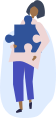Chemistry Homework Help

Get step-by-step solutions from expert tutors.

## How it works?Type, take a picture, or paste your question with all necessary details to ensure high-quality responses.### Get an answer in less than 24hrs

Once our tutors receive the question, they'll begin. You'll get an email once the solution is ready.### Get Notified

We will send you email and text notification to let you know when your question is answered.

### Browse Homework Solutions

58 solutions

Bohr and Balmer Equations

#### Q. An astrophysicist working at an observatory is interested in finding clouds of hydrogen in the galaxy. Usually, hydrogen is detected by looking for Balmer series of spectral lines in the visible spectrum. Unfortunately, the instrument that detects hydrogen emission spectra at this particular observatory is not working very well and only detects spectra in the infrared region of electromagnetic radiation. Therefore, the astrophysicist decides to check for hydrogen by looking at the Paschen series, which produces spectral lines in the infrared part of spectrum. The Paschen series describes the wavelengths of light emitted by the decay of electrons from higher orbits to the n = 3 level. What wavelength λ should the astrophysicist look for to detect a transition of an electron from the n = 7 to the n = 3 level? Express your answer with the appropriate units.

Solved • Feb 13, 2020

Bohr and Balmer Equations

#### Q. Calculate the frequency of the light emitted when an electron in a hydrogen atom makes each of the following transitions. n = 5 → n = 1 Express the frequency in inverse seconds.

Solved • Feb 8, 2020

Bohr and Balmer Equations

#### Q. An electron in a hydrogen atom relaxes to the n = 4 level, emitting light of 138 THz. What is the value of n for the level in which the electron originated? Express your answer as an integer.

Solved • Feb 8, 2020

Bohr and Balmer Equations

#### Q. Calculate the frequency of the light emitted when an electron in a hydrogen atom makes each of the following transitions. n = 6 → n = 5 Express the frequency in inverse seconds.

Solved • Feb 8, 2020

Bohr and Balmer Equations

#### Q. Calculate the frequency of the light emitted when an electron in a hydrogen atom makes each of the following transitions. n = 5 → n = 4 Express the frequency in inverse seconds.

Solved • Feb 8, 2020

Bohr and Balmer Equations

#### Q. Calculate the frequency of the light emitted when an electron in a hydrogen atom makes each of the following transitions. n = 4 → n = 3 Express the frequency in inverse seconds.

Solved • Feb 8, 2020

Bohr and Balmer Equations

#### Q. Shine light of 121.6 nm wavelength on the hydrogen atom and watch what occurs. Pay attention to the change in orbital shape. Repeat the experiment a few times. To what principal quantum level is the electron promoted in every case?

Solved • Feb 5, 2020